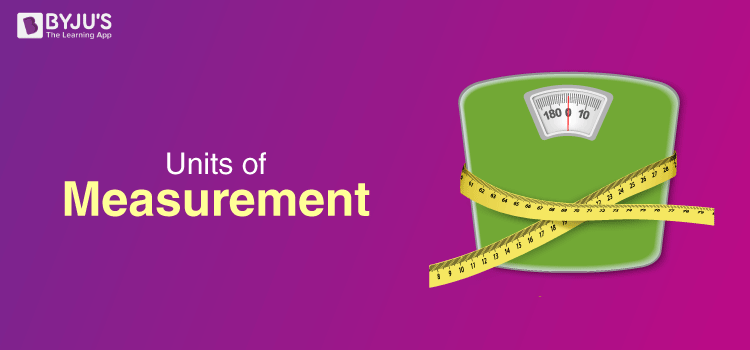# Fundamental and Derived Units of Measurement

## History of measurement

Measurement is one of the fundamental concepts in science. Without the ability to measure it would be difficult for scientists to conduct experiments and form theories. The word “measurement” comes from the Greek word “metron,” which means “limited proportion.”

Measurement is a technique in which the properties of an object are determined by comparing them to a standard.

Measurement requires tools to provide scientists with a quantity. A quantity describes how much of something there is and how many there are. Scientists use a system of measurement still commonly referred to as the “metric system.”  It was the first standardised system of measurement, developed in France in the 1790s. Today, this form is the standard form of measurement in every country except the United States.## The Seven Base Units of Measurement

• Length – Metre (m)

It is defined as the length of the path travelled by light in an interval of exactly

$$\begin{array}{l} \frac {1}{299 792 458}\,\,s.\end{array}$$
It is based on the fundamental quantity, the speed of light in a vacuum which is c = 299,792,458 m/s.

• Time – Second (s)

It is the time taken by 9,192,631,770 periods of oscillations of the light emitted by a caesium -133 atom corresponding to the transition between two hyper-fine levels of the ground state. This is determined by using highly precise atomic clocks.

• Mass – Kilogram (kg)

It is the mass of a prototype platinum-iridium cylinder kept at the International Bureau of Weights and Measures in Paris, France. Copies of this cylinder are kept by many countries which use them to standardise and compare weights.

• Electric current – Ampere (A)

The constant current which, if maintained in two straight parallel conductors of infinite length and negligible circular cross-section when placed 1 m apart in vacuum, would produce a force equal to 2 x 10-7 newton per metre of length between these conductors. While, it may appear that electric charge should have been used as a base unit, measuring current is far easier and hence is chosen as the standard base unit.

• Temperature – Kelvin (K)

The SI unit of temperature is Kelvin. It is exactly 1/(273.16) of the thermodynamic temperature of the triple point of water. The triple point of water is a fixed temperature and pressure at which the solid, liquid and gaseous states can exist at the same time.

• Amount of a substance – Mole (mol)
The mole is the amount of substance which contains as many entities as there are atoms in 0.012 kg of carbon-12. A mole contains an Avogadro number of entities. Check out our chemistry articles to know more about the Avogadro number.

• Luminous Intensity – Candela (cd)

It is the luminous intensity of a source that emits radiation of a constant frequency of 540 x 1012 Hz with a radiant intensity of 1/683  Watt per steradian in any given direction.

### Derived Units

The units of measurement derived from the seven base units specified by the International System of Units are known as SI derived units. They are either dimensionless or can be expressed as a product of one or more of the base units, possibly scaled by an appropriate power of exponentiation. The names of SI derived units, when written in full, are always in lowercase. However, the symbols for units named after persons are written with an uppercase initial letter. For example, the symbol for hertz is “Hz”, but the symbol for metre is “m.” In the table below, we have given a list of derived units.

 Name Symbol Quantity hertz Hz Frequency radian rad angle newton N force, weight farad F electrical capacitance ohm Ω electrical resistance, impedance, reactance weber Wb magnetic flux degree Celsius °C temperature relative to 273.15 K becquerel Bq radioactivity (decays per unit time)

## Frequently Asked Questions – FAQs

Q1

### What is measurement?

Measurement is a technique in which properties of an object are determined by comparing them to a standard.
Q2

### Define a unit.

Any given physical quantity is measured in comparison to a basic unit that is arbitrarily chosen, internationally accepted. The result of a measurement is expressed by a number which is accompanied by a unit.
Q3

### What are significant figures ?

Significant figures can be defined as important numbers between 0 and 9 that are used in the coefficient of an expression that explains the precision of the expression. These figures are obtained by rounding off the expression after the calculations are executed on them.
Q4

### Define supplementary units.

Supplementary units are dimensionless physical quantities that are used along with fundamental units.
Q5

### How is force measured in a metric system?

In the metric system, force is measured in kilograms and is denoted by the symbol kgf.
Test your Knowledge on units of measurement